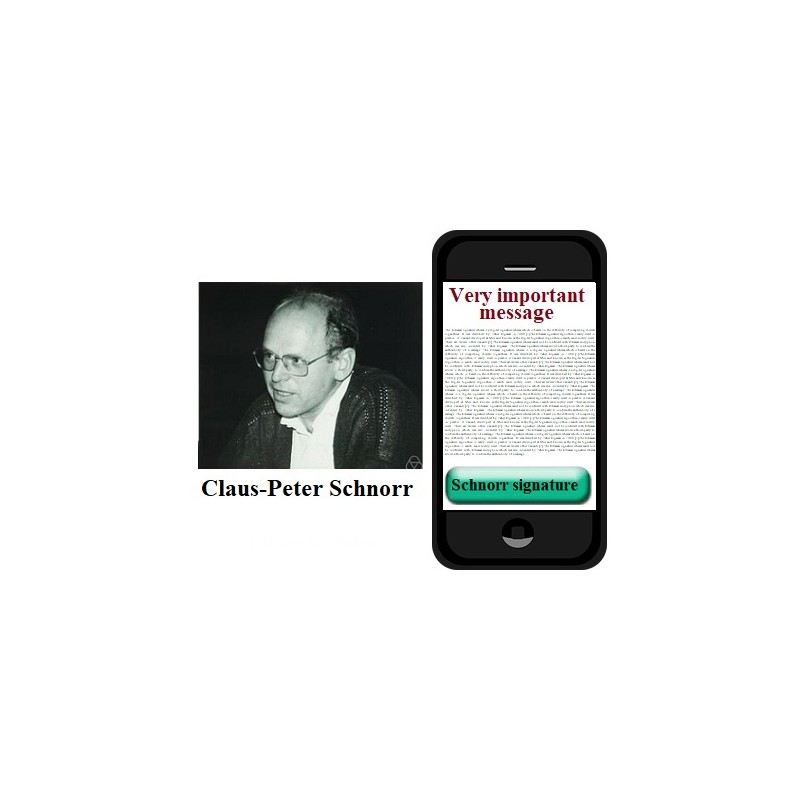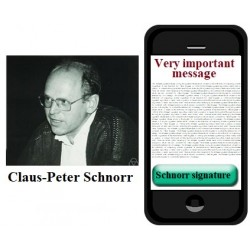•# Schnorr e-signature

€3.00
Tax included

Schnorr e-signature scheme.

Quantity

This protocol is executed between the Student Alice (you) and Mentor Bob. Alice and Bob wants to congratulate each other with birthday. But they don’t know the dates. They want to send to each other these dates and to be sure of their validity to sign them using Schnorr signature.

Parameter values sent by Alice are included in brackets [ ] and must be entered in the corresponding input fields.

The following functions are used in the protocol:

>> genstrongprime(bl)

>> mod_exp(g,x,p)

>> randi(z)

1. Generate strong prime number p of 28 bits length using Octave function >> genstrongprime(28) and find a generator g of multiplicative group Zp*={1,2,3, … p-1}.

Send Public Parameters PP = [p, g] to the Mentor.

1. Ok, generate at random private key PrK=x in the interval 1<x<p-1 and compute public key  PuKA=a=gx mod p. Send [a] to the Mentor to check if your public key is included in his data base previuosly.

1. Ok, look at your birth documents and create a message m1 consisting of date and approximately an hour of your birth m1=YYYYMMDDhh. Choose k=randi(25) and compute r1=gk mod p. Using H-function H26 compute h1=H26(r1||m1). Compute s1=k-xh1mod (p-1). Send message m1 and signature parameters [m1,r1,s1] to the Mentor.

1. Ok, Mentor sends you message m=…..  and his signature parameters (r=….., s=…..) together with his public key PuKA=b=….. . Compute h=H26(r||m) and verify his signature by computing verification parameters V=gsah mod p. To what signature’s parameter corresponds V? Send [V] to the Mentor.

2962 Items